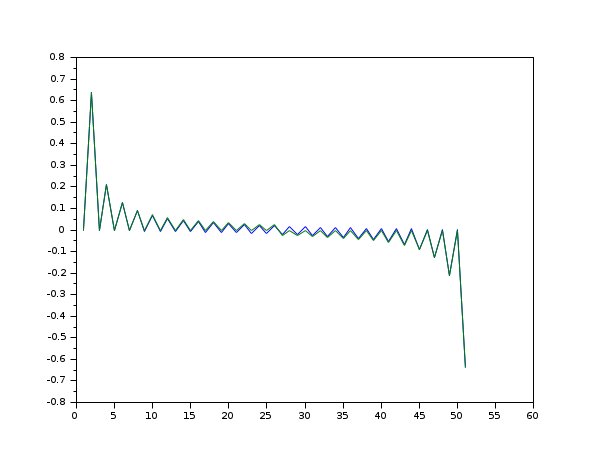Scilab Home page | Wiki | Bug tracker | Forge | Mailing list archives | ATOMS | File exchange
Change language to: English - Português - 日本語 - Русский

Please note that the recommended version of Scilab is 6.0.2. This page might be outdated.
See the recommended documentation of this function

Aide de Scilab >> Traitement du Signal > hilbert

# hilbert

Discrete-time analytic signal computation of a real signal using Hilbert transform

### Calling Sequence

`x=hilbert(xr)`

### Arguments

xr

real vector : the real signal samples

x

Complex vector: the discrete-time analytic signal.

### Description

Returns theanalytic signal, from a real data sequence.

The analytic signal `x= xr + i*xi` has a real part, `xr`, which is the original data, and an imaginary part, `xi`, which contains the Hilbert transform. The imaginary part is a version of the original real sequence with a 90° phase shift.

### References

`http://ieeexplore.ieee.org/iel5/78/16975/00782222.pdf?arnumber=782222`

Marple, S.L., "Computing the discrete-time analytic signal via FFT," IEEE Transactions on Signal Processing, Vol. 47, No.9 (September 1999), pp.2600-2603

• window — compute symmetric window of various type
• hil — FIR approximation to a Hilbert transform filter

### Examples

```//compare the discrete-time analytic signal imaginary part of the impulse real signal
// with the FIR approximation of the Hilbert transform filter
m=25;
n=2*m+1;
y=hilbert(eye(n,1));
h=hilb(n)';
h=[h((m+1):\$);h(1:m)];
plot([imag(y) h])```